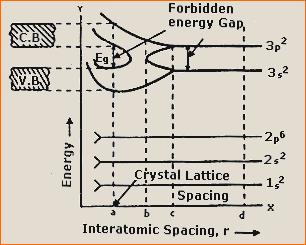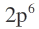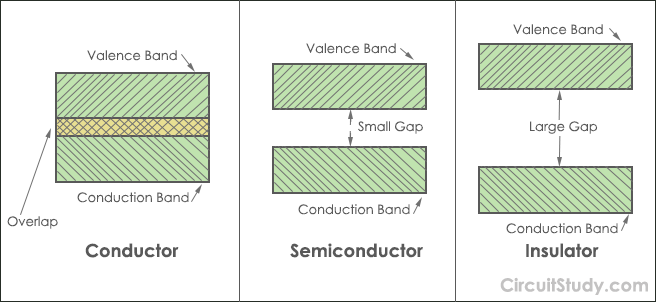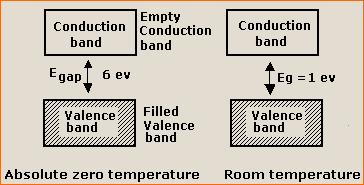# Band theory of Solids

Band Theory of Solids
Energy Band theory is used to study the behaviour of solids as conductors (metals), insulators and semiconductors.
Electrons of each isolated atom have discrete energy levels. When two similar atoms are brought closer, then there is an interaction between the valence electrons of these two. This interaction causes a splitting of each individual energy level into two slightly different energy levels. The atoms in a solid are so close to each other that the energy levels produced after splitting due to interaction between the various atoms will be so close to each other that they appear as continuous. These closely spaced energy levels form an energy band. If the energy band contains the numbers of electrons equal to the number of electrons permitted by exclusion principle, then the energy band is said to be completely filled.
In a completely filled band there is no free electron for the conduction of current. All energy bands above this filled energy band are empty.
How these bands Form?
Consider a silicon (Si) crystal having N atoms.
• The electronic configuration of Silicon (Z = 14) is.
• The energy levels of electrons in the silicon atom are shown in Figure 1.
• The levels 1s, 2s, 2p and 3s are completely filled and the level 3p contains only 2 electrons whereas it can accommodate 6 electrons.
• In a silicon crystal, there are 14N electrons. For each silicon atom, there are two states in energy level 1s. So there are 2N states in energy level 1s, 2N states in energy level 2s, 6N states in energy level 2p, 2N states in energy level 3s and 6N states in 3p energy level of a silicon crystal. In 3p energy level only 2N states are filled and 4N states are empty.
What happens when inter atomic spacing (r) decreases?Figure 1

 1 at r = d > > a (where a = crystal lattice spacing) i.e. when interatomic spacing is very large, Each atom in the silicon crystal behaves independently and has discrete energy levels. 2 at r = c The interaction among valence electrons of N atoms splits 3s and 3p levels into large number of closely spaced energy levels where the energy of an electron may be slightly less or more than the energy of an electron in an isolated atom. Thus two bands corresponding to 3s and 3p states are formed. As the inter atomic spacing (r) is further decreased, the energy bands corresponding to 3s and 3p states spread more and hence energy gap between these bands decreases. The levels 1s², 2s² andlie in the interior of an atom and hence cannot be influenced by other atoms. 3 at r = b The 3s and 3p bands overlap and the energy gap between them disappears. In this case all 8N levels (2N corresponding to 3s energy level and 6N corresponding to 3p energy level) are now continuously distributed. Out of these 8N levels, 4N levels are filled and 4N are empty. 4 at r = a i.e. the equilibrium separation. The filled and unfilled energy levels are separated by an energy gap called forbidden energy gap. The energy of forbidden energy gap is denoted by Eg. The lower filled energy band is called valence band and the upper unfilled energy band is called conduction band.
• Valence band : The energy band occupied by valence electrons is known as valence band.The electrons in the outermost orbit of an atom are called valence electrons. In a normal atom, valence band has the electrons of highest energy. This band may be totally or partially filled.
• Conduction band : The band occupied by the electrons coming from the valence band is called conduction band.It is either empty or partially filled and lies above the valence band.
Generally, insulators have empty conduction band while conductors possess a partially filled conduction band.
• Forbidden energy gap : The separation between conduction band and valence band on the energy level diagram is known as forbidden energy gap or the minimum energy required by an electron to jump from valence band to the conduction band is called forbidden energy gap.
Note:
1. No electron of a solid can stay in a forbidden energy gap as there is no allowed energy state in this region. The width of the forbidden energy gap is a measure of the bondage of valence electrons to the atom.
2. The greater the energy gap, more tightly the valence electrons are bound to the nucleus. In order to transit an electron from valence band to the conduction band (i.e. to make the valence electron free) an external energy equal to the forbidden energy gap must be supplied.
A solid can behave as a conductor, insulator or a semiconductor depending on the width of the forbidden energy band. A material, therefore, can be classified as a conductor, insulator, or a semiconductor on the basis of its energy band diagram.
(A) Conductors
(B) Insulators
(C) SemiconductorsS.No. Conductors Insulators Semiconductors 1. The valence and conduction bands overlap on each other. The valence band is completely filled with electrons and the conduction band is empty. Semiconductors are the materials in which the forbidden energy gap between the valence band and the conduction band is very small. 2. There is no forbidden energy gap. Both the bands are separated by a forbidden energy gap of about 6-7 eV. Both the bands are separated by a forbidden energy gap less than 3 eV. 3. As there is a large number of conduction electrons, so conductors or metals are good conductors of electricity. Insulator is a bad conductor of electricity. At 0 K, the electrons in the valence band do not have sufficient energy to jump to the conduction band and hence semiconductor behaves as an insulator at 0 K. But at room temperature, some of the valence electrons have sufficient thermal energy to jump to the conduction band. Hence,semiconductor may conduct at room temperature. 4. Silver, copper, aluminium, etc. are the example of insulators. Alumina, glass, nylon, etc. are the example of insulators. Germanium, silicon, etc. are the example of insulators. 5. Conductivity increases with increase in temperature. Conductivity increases but very slowly with increase in temperature. Conductivity increases with increase in temperature. 6. Resistivity decreases with increase in temperature. Resistivity decreases  very slowly with increase in temperature. Resistivity decreases with increase in temperature.

Effect of temperature in Semiconductor:This site uses Akismet to reduce spam. Learn how your comment data is processed.

error: Content is protected !!